Next: Method of Stationary Phase Up: Wave Propagation in Uniform Previous: Wave-Front Propagation

# Sommerfeld Precursor

Consider the situation immediately after the arrival of the signal: that is, when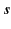is small and positive. Let us start from Equation (887), which can be written in the form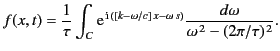(890)

We can deform the original path of integration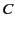into a large semi-circle of radius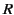in the upper half-plane, plus two segments of the real axis, as shown in Figure 9. Because of the denominator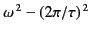, the integrand tends to zero as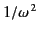on the real axis. We can add the path in the lower half-plane that is shown as a dotted line in the figure, because if the radius of the semi-circular portion of this lower path is increased to infinity then the integrand vanishes exponentially as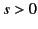. Therefore, we can replace our original path of integration by the entire circle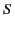. Thus,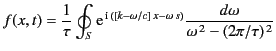(891)

in the limit that the radius of the circletends to infinity.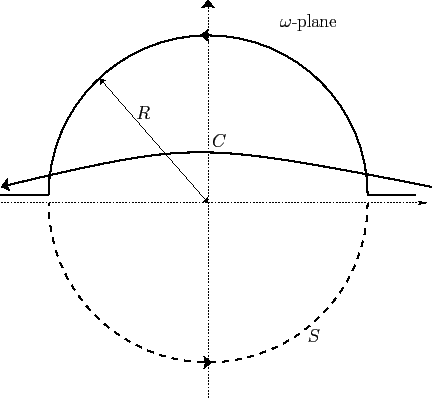The dispersion relation (871) yields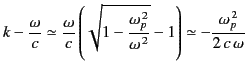(892)

in the limit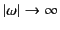. Using the abbreviation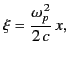(893)

and, henceforth, neglecting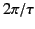with respect to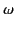, we obtain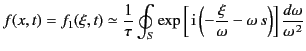(894)

from Equation (892). This expression can also be written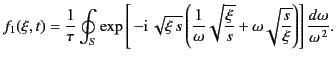(895)

Let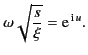(896)

It follows that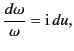(897)

giving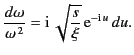(898)

Substituting the angular variable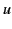forin Equation (896), we obtain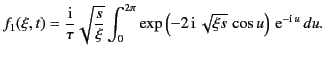(899)

Here, we have taken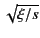as the radius of the circular integration path in the-plane. This is indeed a large radius because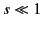. From symmetry, Equation (900) simplifies to give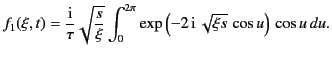(900)

The following mathematical identity is fairly well known,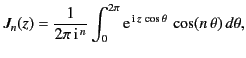(901)

where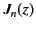is Bessel function of order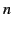. It follows from Equation (901) that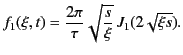(902)

Here, we have made use of the fact that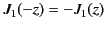.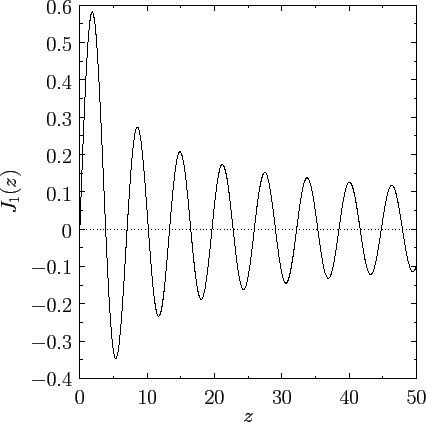The properties of Bessel functions are described in many standard references on mathematical functions (see, for instance, Abramowitz and Stegun). In the small argument limit,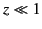, we find that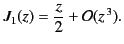(903)

On the other hand, in the large argument limit,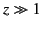, we obtain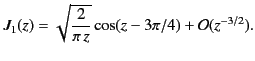(904)

The behavior of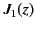is further illustrated in Figure 10.

We are now in a position to make some quantitative statements regarding the signal that first arrives at a depth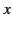within the dispersive medium. This signal propagates at the velocity of light in vacuum, and is called the Sommerfeld precursor. The first important point to note is that the amplitude of the Sommerfeld precursor is very small compared to that of the incident wave (whose amplitude is normalized to unity). We can easily see this because, in deriving Equation (903), we assumed that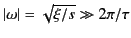on the circular integration path. Because the magnitude of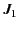is always less than, or of order, unity, it is clear that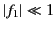. This is a comforting result, because in a naive treatment of wave propagation through a dielectric medium, the wave-front propagates at the group velocity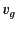(which is less than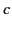) and, therefore, no signal should reach a depthwithin the medium before time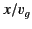. We are finding that there is, in fact, a precursor that arrives at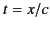, but that this signal is fairly weak. Note from Equation (894) that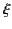is proportional to. Consequently, the amplitude of the Sommerfeld precursor decreases as the inverse of the distance traveled by the wave-front through the dispersive medium [because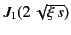attains its maximum value when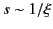]. Thus, the Sommerfeld precursor is likely to become undetectable after the wave has traveled a long distance through the medium.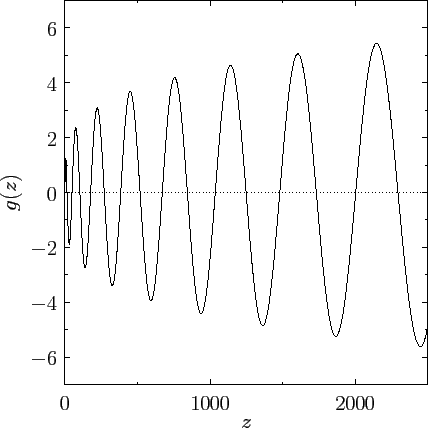Equation (903) can be written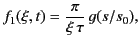(905)

where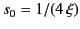, and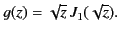(906)

The normalized Sommerfeld precursor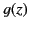is shown in Figure 11. It can be seen that both the amplitude and the oscillation period of the precursor gradually increase. The roots of[i.e., the solutions of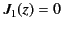] are spaced at distances of approximately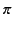apart. Thus, the time interval for the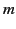th half period of the precursor is approximately given by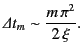(907)

Note that the initial period of oscillation,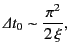(908)

is extremely small compared to the incident period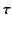. Moreover, the initial period of oscillation is completely independent of the frequency of the incident wave. In fact,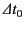depends only on the propagation distance, and the dispersive power of the medium. The period also decreases with increasing distance,, traveled by the wave-front though the medium. So, when visible radiation is incident on a dispersive medium, it is quite possible for the first signal detected well inside the medium to lie in the X-ray region of the electromagnetic spectrum.Next: Method of Stationary Phase Up: Wave Propagation in Uniform Previous: Wave-Front Propagation
Richard Fitzpatrick 2014-06-27